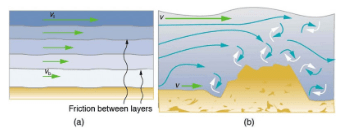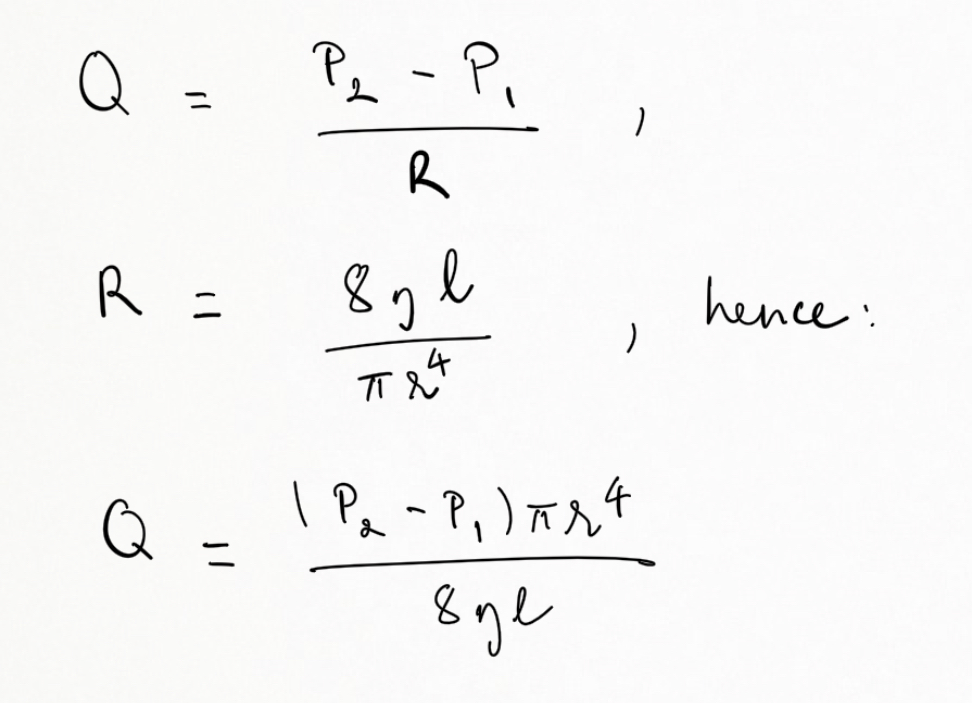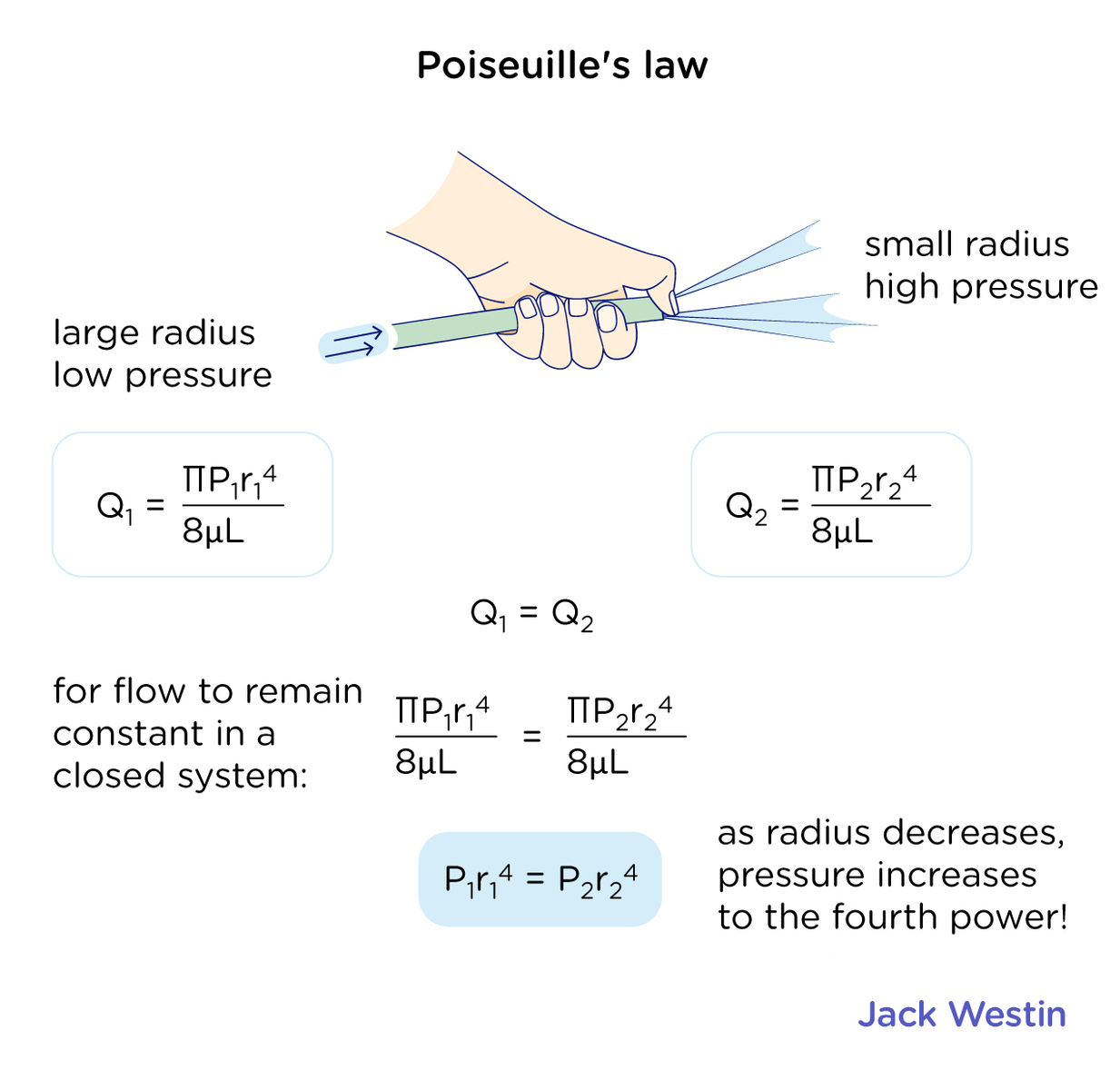Complimentary 1-hour tutoring consultation

MCAT Content / Fluids / Viscosity Poiseuille Flow

### Viscosity: Poiseuille Flow

Topic: Fluids

Viscosity is a quantity expressing the magnitude of internal friction in a fluid. Poiseuille’s law describes the pressure-induced, laminar flow through a tube.

When you pour yourself a glass of juice, the liquid flows freely and quickly. But when you pour syrup on your pancakes, that liquid flows slowly and sticks to the pitcher. The difference is fluid friction, both within the fluid itself and between the fluid and its surroundings. We call this property of fluids viscosity. Juice has low viscosity, whereas syrup has high viscosity.

Viscosity of a fluid is measured and defined based on laminar flow. Laminar flow (a) is characterized by the smooth flow of the fluid in layers that do not mix. Turbulent flow (b), or turbulence, is characterized by eddies and swirls that mix layers of fluid together.Viscosity of a fluid is measured by laminar flow of fluid between two plates of area A:

η = FL/vA

Where F is the force required to keep the top plate in the figure below moving at a constant velocity v,  L is distance between the plates and A is the area of the plate. The SI unit of viscosity is (N/m2)s or Pa⋅s.Poiseuille flow is pressure-induced flow in a long duct, usually a pipe. It is assumed that there is a laminar flow of an incompressible fluid of viscosity η. Flow rate Q is in the direction from high to low pressure. The greater the pressure differential between two points (P2 – P1), the greater the flow rate. This relationship can be stated asThis equation describes laminar flow through a tube. It is sometimes called Poiseuille’s law for laminar flow, or simply Poiseuille’s law.Practice Questions

Understanding cardiac pressure-volume curves

MCAT Official Prep (AAMC)

Section Bank C/P Section Question 100

Practice Exam 3 C/P Section Passage 9 Question 50

Key Points

• Laminar flow: smooth flow of the fluid in layers that do not mix. Turbulent flow: swirls that mix layers of fluid together.

• Viscosity is a quantity expressing the magnitude of internal friction in a fluid, defined based on laminar flow.

• Poiseuille’s law describes the pressure-induced, laminar flow through a tube.

Key Terms

Laminar: A type of fluid flow in which layers do not mix

Turbulence: A fluid flow in which layers mix together via eddies and swirls

Viscosity: The friction in a fluid, defined in terms of the friction between layers

Poiseuille’s law for resistance: The resistance to laminar flow of an incompressible fluid in a tube: R = 8ηl/πr4

Poiseuille’s law: The rate of laminar flow of an incompressible fluid in a tube: Q = (P2 − P1)πr4/8ηl

Billing Information